# TuningGoal.LoopShape class

Package: TuningGoal

Target loop shape for control system tuning

## Description

Use `TuningGoal.LoopShape` to specify a target gain profile (gain as a function of frequency) of an open-loop response. `TuningGoal.LoopShape` constrains the open-loop, point-to-point response (L) at a specified location in your control system. Use this tuning goal for control system tuning with tuning commands, such as `systune` or `looptune`.

When you tune a control system, the target open-loop gain profile is converted into constraints on the inverse sensitivity function inv(S)  = (I + L) and the complementary sensitivity function T = 1–S. These constraints are illustrated for a representative tuned system in the following figure.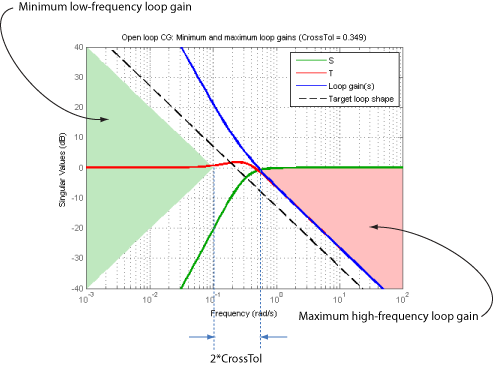Where L is much greater than 1, a minimum gain constraint on inv(S) (green shaded region) is equivalent to a minimum gain constraint on L. Similarly, where L is much smaller than 1, a maximum gain constraint on T (red shaded region) is equivalent to a maximum gain constraint on L. The gap between these two constraints is twice the `CrossTol` parameter, which specifies the frequency band where the loop gain can cross 0 dB.

For multi-input, multi-output (MIMO) control systems, values in the gain profile greater than 1 are interpreted as minimum performance requirements. Such values are lower bounds on the smallest singular value of the open-loop response. Gain profile values less than one are interpreted as minimum roll-off requirements, which are upper bounds on the largest singular value of the open-loop response. For more information about singular values, see `sigma`.

Use `TuningGoal.LoopShape` when the loop shape near crossover is simple or well understood (such as integral action). To specify only high gain or low gain constraints in certain frequency bands, use `TuningGoal.MinLoopGain` and `TuningGoal.MaxLoopGain`. When you do so, the software determines the best loop shape near crossover.

## Construction

```Req = TuningGoal.LoopShape(location,loopgain)``` creates a tuning goal for shaping the open-loop response measured at the specified location. The magnitude of the single-input, single-output (SISO) transfer function `loopgain` specifies the target open-loop gain profile. You can specify the target gain profile (maximum gain across the I/O pair) as a smooth transfer function or sketch a piecewise error profile using an `frd` model.

`Req = TuningGoal.LoopShape(location,loopgain,crosstol)` specifies a tolerance on the location of the crossover frequency. `crosstol` expresses the tolerance in decades. For example, `crosstol` = 0.5 allows gain crossovers within half a decade on either side of the target crossover frequency specified by `loopgain`. When you omit `crosstol`, the tuning goal uses a default value of 0.1 decades. You can increase `crosstol` when tuning MIMO control systems. Doing so allows more widely varying crossover frequencies for different loops in the system.

`Req = TuningGoal.LoopShape(location,wc)` specifies just the target gain crossover frequency. This syntax is equivalent to specifying a pure integrator loop shape, `loopgain = wc/s`.

`Req = TuningGoal.LoopShape(location,wcrange)` specifies a range for the target gain crossover frequency. The range is a vector of the form `wcrange` = `[wc1,wc2]`. This syntax is equivalent to using the geometric mean `sqrt(wc1*wc2)` as `wc` and setting `crosstol` to the half-width of `wcrange` in decades. Using a range instead of a single `wc` value increases the ability of the tuning algorithm to enforce the target loop shape for all loops in a MIMO control system.

### Input Arguments

 `location` Location where the open-loop response shape to be constrained is measured, specified as a character vector or cell array of character vectors that identify one or more locations in the control system to tune. What locations are available depends on what kind of system you are tuning:If you are tuning a Simulink® model of a control system, you can use any linear analysis point marked in the model, or any linear analysis point in an `slTuner` interface associated with the Simulink model. Use `addPoint` to add analysis points to the `slTuner` interface. For example, if the `slTuner` interface contains an analysis point `u`, you can use `'u'` to refer to that point when creating tuning goals. Use `getPoints` to get the list of analysis points available in an `slTuner` interface to your model.If you are tuning a generalized state-space (`genss`) model of a control system, you can use any `AnalysisPoint` location in the control system model. For example, the following code creates a PI loop with an analysis point at the plant input `'u'`. ```AP = AnalysisPoint('u'); G = tf(1,[1 2]); C = tunablePID('C','pi'); T = feedback(G*AP*C,1); ```When creating tuning goals, you can use `'u'` to refer to the analysis point at the plant input. Use `getPoints` to get the list of analysis points available in a `genss` model. The loop shape requirement applies to the point-to-point open-loop transfer function at the specified location. That transfer function is the open-loop response obtained by injecting signals at the location and measuring the return signals at the same point. If `location` specifies multiple locations, then the loop-shape requirement applies to the MIMO open-loop transfer function. `loopgain` Target open-loop gain profile as a function of frequency. You can specify `loopgain` as a smooth SISO transfer function (`tf`, `zpk`, or `ss` model). Alternatively, you can sketch a piecewise gain profile using a `frd` model or the `makeweight` function. When you do so, the software automatically maps your specified gain profile to a `zpk` model whose magnitude approximates the desired gain profile. Use `viewGoal(Req)` to plot the magnitude of that `zpk` model. For multi-input, multi-output (MIMO) control systems, values in the gain profile greater than 1 are interpreted as minimum performance requirements. These values are lower bounds on the smallest singular value of `L`. Gain profile values less than one are interpreted as minimum roll-off requirements, which are upper bounds on the largest singular value of `L`. For more information about singular values, see `sigma`. If you are tuning in discrete time (that is, using a `genss` model or `slTuner` interface with nonzero `Ts`), you can specify `loopgain` as a discrete-time model with the same `Ts`. If you specify `loopgain` in continuous time, the tuning software discretizes it. Specifying the loop shape in discrete time gives you more control over the loop shape near the Nyquist frequency. `crosstol` Tolerance in the location of crossover frequency, in decades. specified as a scalar value. For example, `crosstol` = 0.5 allows gain crossovers within half a decade on either side of the target crossover frequency specified by `loopgain`. Increasing `crosstol` increases the ability of the tuning algorithm to enforce the target loop shape for all loops in a MIMO control system. Default: 0.1 `wc` Target crossover frequency, specified as a positive scalar value. Express `wc` in units of rad/`TimeUnit`, where `TimeUnit` is the `TimeUnit` property of the control system model you are tuning. `wcrange` Range for target crossover frequency, specified as a vector of the form `[wc1,wc2]`. Express `wc` in units of rad/`TimeUnit`, where `TimeUnit` is the `TimeUnit` property of the control system model you are tuning.

## Properties

 `LoopGain` Target loop shape as a function of frequency, specified as a SISO `zpk` model. The software automatically maps the input argument `loopgain` onto a `zpk` model. The magnitude of this `zpk` model approximates the desired gain profile. Use `viewGoal(Req)` to plot the magnitude of the `zpk` model `LoopGain`. `CrossTol` Tolerance on gain crossover frequency, in decades. The initial value of `CrossTol` is set by the `crosstol` input when you create the tuning goal. Default: `0.1` `Focus` Frequency band in which tuning goal is enforced, specified as a row vector of the form `[min,max]`. Set the `Focus` property to limit enforcement of the tuning goal to a particular frequency band. Express this value in the frequency units of the control system model you are tuning (rad/`TimeUnit`). For example, suppose `Req` is a tuning goal that you want to apply only between 1 and 100 rad/s. To restrict the tuning goal to this band, use the following command:`Req.Focus = [1,100];` Default: `[0,Inf]` for continuous time; `[0,pi/Ts]` for discrete time, where `Ts` is the model sample time. `Stabilize` Stability requirement on closed-loop dynamics, specified as 1 (`true`) or 0 (`false`). When `Stabilize` is `true`, this requirement stabilizes the specified feedback loop, as well as imposing gain or loop-shape requirements. Set `Stabilize` to `false` if stability for the specified loop is not required or cannot be achieved. Default: 1 (`true`) `LoopScaling` Toggle for automatically scaling loop signals, specified as `'on'` or `'off'`. In multi-loop or MIMO control systems, the feedback channels are automatically rescaled to equalize the off-diagonal terms in the open-loop transfer function (loop interaction terms). Set `LoopScaling` to `'off'` to disable such scaling and shape the unscaled open-loop response. Default: `'on'` `Location` Location at which the open-loop response shape to be constrained is measured, specified as a cell array of character vectors that identify one or more analysis points in the control system to tune. For example, if `Location = {'u'}`, the tuning goal evaluates the open-loop response measured at an analysis point `'u'`. If `Location = {'u1','u2'}`, the tuning goal evaluates the MIMO open-loop response measured at analysis points `'u1'` and `'u2'`. The initial value of the `Location` property is set by the `location` input argument when you create the tuning goal. `Models` Models to which the tuning goal applies, specified as a vector of indices. Use the `Models` property when tuning an array of control system models with `systune`, to enforce a tuning goal for a subset of models in the array. For example, suppose you want to apply the tuning goal, `Req`, to the second, third, and fourth models in a model array passed to `systune`. To restrict enforcement of the tuning goal, use the following command: `Req.Models = 2:4;` When `Models = NaN`, the tuning goal applies to all models. Default: `NaN` `Openings` Feedback loops to open when evaluating the tuning goal, specified as a cell array of character vectors that identify loop-opening locations. The tuning goal is evaluated against the open-loop configuration created by opening feedback loops at the locations you identify. If you are using the tuning goal to tune a Simulink model of a control system, then `Openings` can include any linear analysis point marked in the model, or any linear analysis point in an `slTuner` interface associated with the Simulink model. Use `addPoint` to add analysis points and loop openings to the `slTuner` interface. Use `getPoints` to get the list of analysis points available in an `slTuner` interface to your model. If you are using the tuning goal to tune a generalized state-space (`genss`) model of a control system, then `Openings` can include any `AnalysisPoint` location in the control system model. Use `getPoints` to get the list of analysis points available in the `genss` model. For example, if `Openings = {'u1','u2'}`, then the tuning goal is evaluated with loops open at analysis points `u1` and `u2`. Default: `{}` `Name` Name of the tuning goal, specified as a character vector. For example, if `Req` is a tuning goal: `Req.Name = 'LoopReq';` Default: `[]`

## Examples

collapse all

Create a target gain profile requirement for the following control system. Specify integral action, gain crossover at 1, and a roll-off requirement of 40 dB/decade.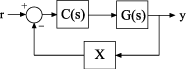The requirement should apply to the open-loop response measured at the `AnalysisPoint` block `X`. Specify a crossover tolerance of 0.5 decades.

```LS = frd([100 1 0.0001],[0.01 1 100]); Req = TuningGoal.LoopShape('X',LS,0.5);```

The software converts `LS` into a smooth function of frequency that approximates the piecewise-specified requirement. Display the requirement using `viewGoal`.

`viewGoal(Req)`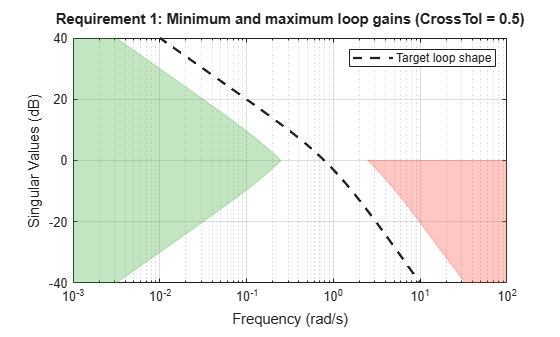The green and red regions indicate the bounds for the inverse sensitivity, `inv(S) = 1-G*C`, and the complementary sensitivity, `T = 1-S`, respectively. The gap between these regions at 0 dB gain reflects the specified crossover tolerance, which is half a decade to either side of the target loop crossover.

When you use `viewGoal(Req,CL)` to validate a tuned closed-loop model of this control system, `CL`, the tuned values of `S` and `T` are also plotted.

Create separate loop shape requirements for the inner and outer loops of the following control system.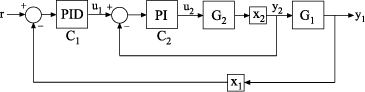For the inner loop, specify a loop shape with integral action, gain crossover at 1, and a roll-off requirement of 40 dB/decade. Additionally, specify that this loop shape requirement should be enforced with the outer loop open.

```LS2 = frd([100 1 0.0001],[0.01 1 100]); Req2 = TuningGoal.LoopShape('X2',LS2); Req2.Openings = 'X1';```

Specifying `'X2'` for the `location` indicates that `Req2` applies to the point-to point, open-loop transfer function at the location `X2`. Setting `Req2.Openings` indicates that the loop is opened at the analysis point `X1` when `Req2` is enforced.

By default, `Req2` imposes a stability requirement on the inner loop as well as the loop shape requirement. In some control systems, however, inner-loop stability might not be required, or might be impossible to achieve. In that case, remove the stability requirement from `Req2` as follows.

`Req2.Stabilize = false;`

For the outer loop, specify a loop shape with integral action, gain crossover at 0.1, and a roll-off requirement of 20 dB/decade.

```LS1 = frd([10 1 0.01],[0.01 0.1 10]); Req1 = TuningGoal.LoopShape('X1',LS1);```

Specifying `'X1'` for the `location` indicates that `Req1` applies to the point-to point, open-loop transfer function at the location `X1`. You do not have to set `Req1.Openings` because this loop shape is enforced with the inner loop closed.

You might want to tune the control system with both loop shaping requirements `Req1` and `Req2`. To do so, use both requirements as inputs to the tuning command. For example, suppose `CL0` is a tunable `genss` model of the closed-loop control system. In that case, use `[CL,fSoft] = systune(CL0,[Req1,Req2])` to tune the control system to both requirements.

Create a loop-shape requirement for the feedback loop on `'q'` in the Simulink model `rct_airframe2`. Specify that the loop-shape requirement is enforced with the `'az'` loop open.

Open the model.

```open_system('rct_airframe2') ```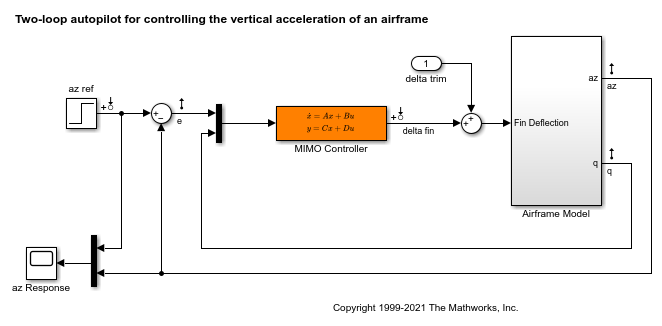Create a loop shape requirement that enforces integral action with a crossover a 2 rad/s for the `'q'` loop. This loop shape corresponds to a loop shape of 2/_s_.

```s = tf('s'); shape = 2/s; Req = TuningGoal.LoopShape('q',shape); ```

Specify the location at which to open an additional loop when enforcing the requirement.

```Req.Openings = 'az'; ```

To use this requirement to tune the Simulink model, create an `slTuner` interface to the model. Identify the block to tune in the interface.

```ST0 = slTuner('rct_airframe2','MIMO Controller'); ```

Designate both `az` and `q` as analysis points in the `slTuner` interface.

```addPoint(ST0,{'az','q'}); ```

This command makes `q` available as an analysis location. It also allows the tuning requirement to be enforced with the loop open at `az`.

You can now tune the model using `Req` and any other tuning requirements. For example:

```[ST,fSoft] = systune(ST0,Req); ```
```Final: Soft = 0.845, Hard = -Inf, Iterations = 51 ```

Create a tuning requirement specifying that the open-loop response of loop identified by `'X'` cross unity gain between 50 and 100 rad/s.

`Req = TuningGoal.LoopShape('X',[50,100]);`

Examine the resulting requirement to see the target loop shape.

`viewGoal(Req)`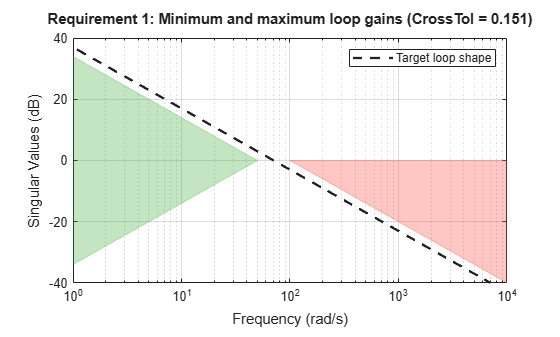The plot shows that the requirement specifies an integral loop shape, with crossover around 70 rad/s, the geometrical mean of the range [50,100]. The gap at 0 dB between the minimum low-frequency gain (green region) and the maximum high-frequency gain (red region) reflects the allowed crossover range [50,100].

## Tips

• This tuning goal imposes an implicit stability constraint on the closed-loop sensitivity function measured at `Location`, evaluated with loops opened at the points identified in `Openings`. The dynamics affected by this implicit constraint are the stabilized dynamics for this tuning goal. The `MinDecay` and `MaxRadius` options of `systuneOptions` control the bounds on these implicitly constrained dynamics. If the optimization fails to meet the default bounds, or if the default bounds conflict with other requirements, use `systuneOptions` to change these defaults.

## Algorithms

When you tune a control system using a `TuningGoal`, the software converts the tuning goal into a normalized scalar value f(x), where x is the vector of free (tunable) parameters in the control system. The software then adjusts the parameter values to minimize f(x) or to drive f(x) below 1 if the tuning goal is a hard constraint.

For `TuningGoal.LoopShape`, f(x) is given by:

`$f\left(x\right)={‖\begin{array}{c}{W}_{S}S\\ {W}_{T}T\end{array}‖}_{\infty }.$`

Here, S = D–1[I – L(s,x)]–1D is the scaled sensitivity function at the specified location, where L(s,x) is the open-loop response being shaped. D is an automatically-computed loop scaling factor. (If the `LoopScaling` property is set to `'off'`, then D = I.) T = S – I is the complementary sensitivity function.

WS and WT are frequency weighting functions derived from the specified loop shape. The gains of these functions roughly match `LoopGain` and `1/LoopGain`, for values ranging from –20 dB to 60 dB. For numerical reasons, the weighting functions level off outside this range, unless the specified loop gain profile changes slope for gains above 60 dB or below –60 dB. Because poles of WS or WT close to s = 0 or s = `Inf` might lead to poor numeric conditioning of the `systune` optimization problem, it is not recommended to specify loop shapes with very low-frequency or very high-frequency dynamics.

To obtain WS and WT, use:

`[WS,WT] = getWeights(Req,Ts)`

where `Req` is the tuning goal, and `Ts` is the sample time at which you are tuning (`Ts = 0` for continuous time). For more information about the effects of the weighting functions on numeric stability, see Visualize Tuning Goals.

## Compatibility Considerations

expand all

Behavior changed in R2016a